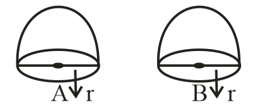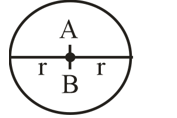#### Write ‘True’ or ‘False’ and justify your answer in the following: Two identical solid hemispheres of equal base radius r cm are stuck together along their bases. The total surface area of the combination is .

It is given that there is two hemispheres of radius r.

Let A and B are two hemispheres of radius r.Join A and B along with their basesNow it is a full sphere of radius r

The total surface area of a sphere

Here we found that the total surface area of the combination is , but not